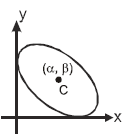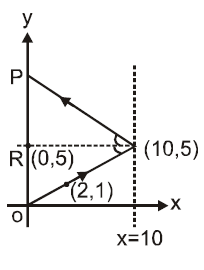# An ellipse has semi-major axes of lengths 2 and semi-minor axis of length 1. It is slipping between the coordinate axes in the first quadrant while maintaining contact with both x-axis and y-axis.Q. When the ordinate of the centre of ellipse is 1, a light ray passing through origin and centre of ellipse strikes the tangent at the vertex of the parabola y2 + 4x – 10y – 15 = 0. The reflected ray cuts y-axis at the pointa)(0, 8)b)(0, 10)c)(0, –8)d)(0, 12)Correct answer is option 'B'. Can you explain this answer? Related Test: Part Test - 5 (JEE Advanced 2020)

## JEE QuestionKarambir Singh Jul 22, 2020
You may use the fact that the product of perpendicular distances of any tangent on ellipse from its foci is
always equal to square of semi-minor axis.
Since the path of centre is x2 + y2 = 5 when y = 1, x = 2.
The incident ray passes through origin and (2, 1) ⇒ its equation is y = 1/2x
Given parabola is (y – 5)2 = – 4 (x – 10)
whose vertex (10, 5) lies on y = 1/2x.
The tangent at the vertex is the line x = 10.
Figure shows that OP = 2 (OR) = 10
so reflected ray cuts y-axis at (0, 10)